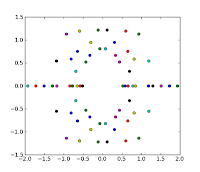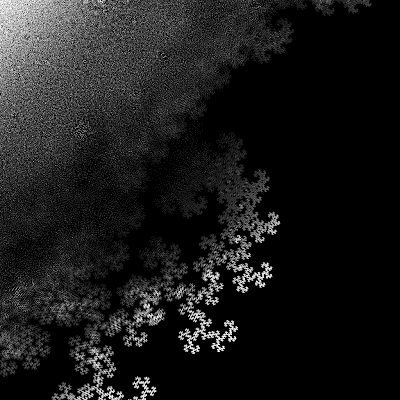### Roots of polinomialsАльбом: Math

Mathworld article about polynomial roots contains an interesting idea. Consider polynomials of some order N with coefficients ±1:

P(x) = xN ± xN-1 ± xN-2 ± ... ± 1.

Apparently, there are 2N-1 different polynomials of order N. What are their roots look like on a complex plane? Picture below shows roots (white points) of all 16 polynomials of the 5th order:But what if we take polynomials of the higher power?.

Picture at the top is the answer. If shows all roots of the all ±1 polynomials of order 21. there is 220*21 = 22'020'096 roots in total, so most of them are indistinguishable. Areas, where roots are denser are brighter. Bright horizontal line in the center, corresponds the real roots.

This root amp do not change appearance significantly with the order: increasing order only makes it more detailed and slightly changes size of the pinned-out black areas on the main circle.

Additionally to the obvious horizontal and vertical symmetry, this image also symmetric relative to the inversion, because if P(x) is a ±1 polynomial of order N, then xNP(1/x) is also ±1 polynomial, and its roots are inverse of the original ones.
The picture has obviously fractal nature, and similar to the IFS fractals. Here is zoom-up of the side:Альбом: Math